# 2. Discrete Fourier Transform.(/25) 1. N-th roots of unity are defined as solutions to the equation:...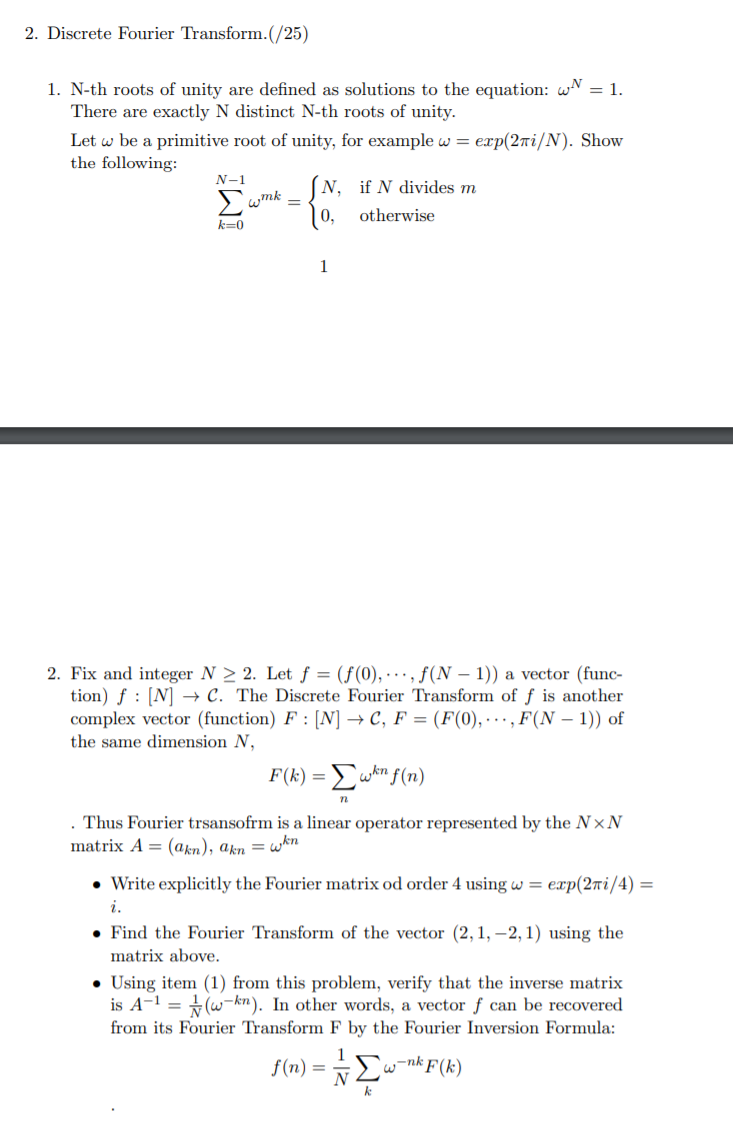2. Discrete Fourier Transform.(/25) 1. N-th roots of unity are defined as solutions to the equation: w = 1. There are exactly N distinct N-th roots of unity. Let w be a primitive root of unity, for example w = exp(2 i/N). Show the following: N, if N divides m k=0 10, otherwise N -1 N wmk 2. Fix and integer N > 2. Let f = (f(0), ..., f(N − 1)) a vector (func- tion) f : [N] → C. The Discrete Fourier Transform of f is another complex vector (function) F: [N] → C, F = (F(0),...,F(N − 1)) of the same dimension N, F(k) = wkn f(n) . Thus Fourier trsansofrm is a linear operator represented by the NxN matrix A= (akn), akn = win • Write explicitly the Fourier matrix od order 4 using w = exp(2mi/4) = • Find the Fourier Transform of the vector (2,1, -2, 1) using the matrix above. • Using item (1) from this problem, verify that the inverse matrix is A-1 = \$w-kn). In other words, a vector f can be recovered from its Fourier Transform F by the Fourier Inversion Formula: f(n) = 1 Çw=nk F(x)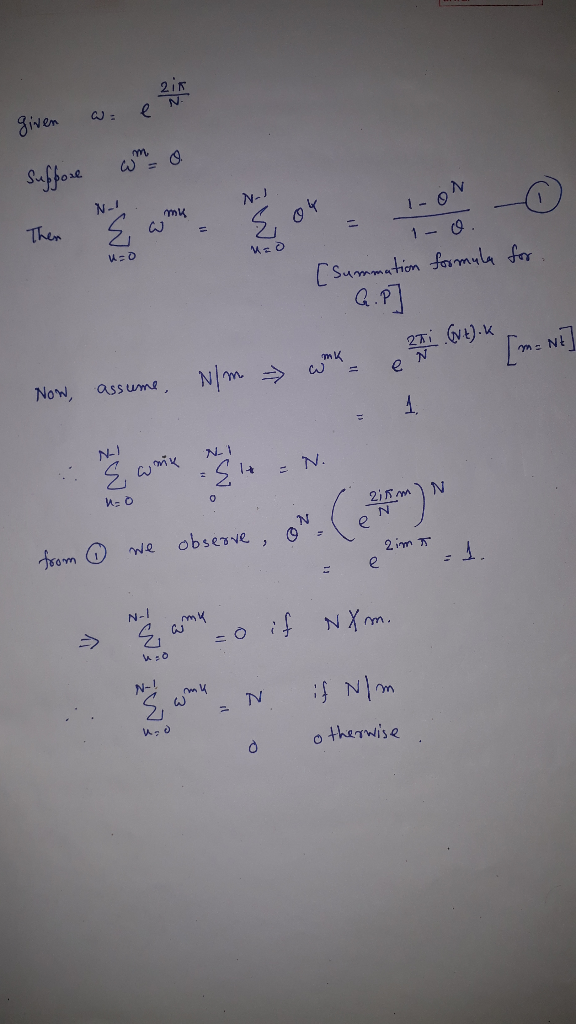#### Earn Coin

Coins can be redeemed for fabulous gifts.

Similar Homework Help Questions
• ### 2TT sinn (1) a) Let x1 [n] = πη Find the Discrete Time Fourier transform of...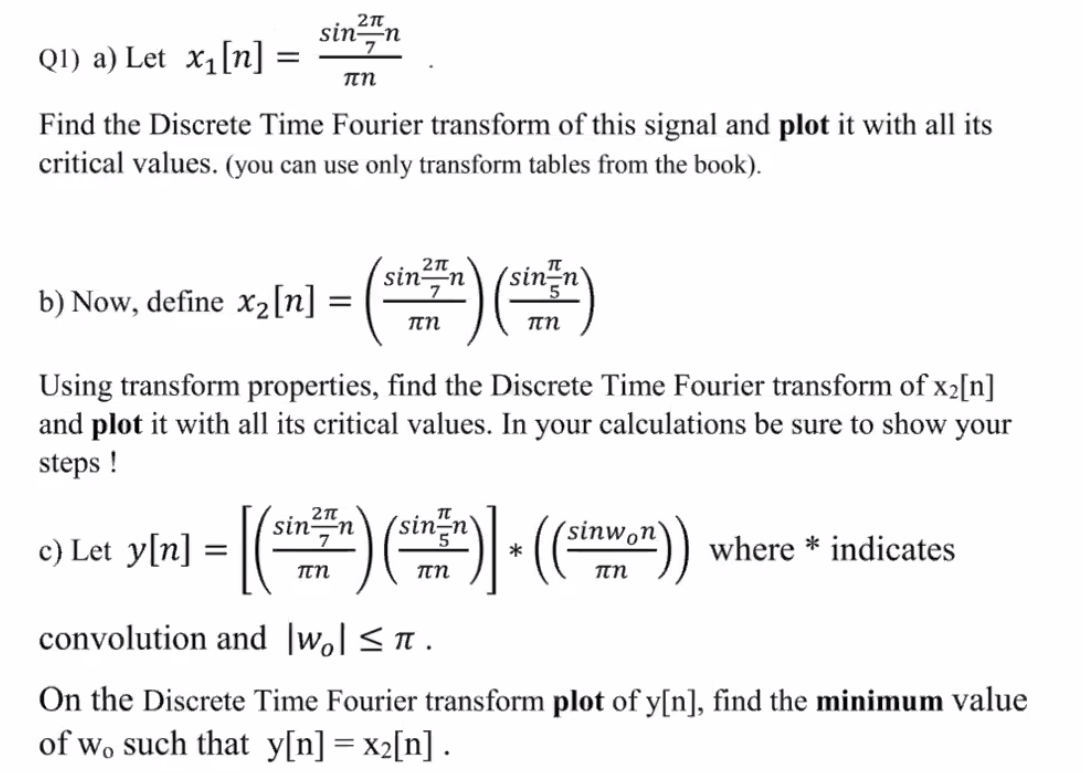2TT sinn (1) a) Let x1 [n] = πη Find the Discrete Time Fourier transform of this signal and plot it with all its critical values. (you can use only transform tables from the book). b) Now, define xzlv) = (**) GHS) Using transform properties, find the Discrete Time Fourier transform of x2[n] and plot it with all its critical values. In your calculations be sure to show your steps ! 2TT sinn sinn sinwon c) Let y[n] [( )...

• ### Show that Discrete Fourier transform matrix W /Vn is a unitary matrix, where e2j/n and i = V-1, W = 1 1 1 1 1 (n-1)...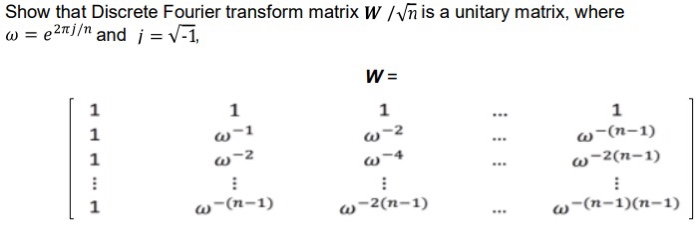Show that Discrete Fourier transform matrix W /Vn is a unitary matrix, where e2j/n and i = V-1, W = 1 1 1 1 1 (n-1) w-2(n-1) w-2 1 z- w~(n-1) -2(n-1) (n-1)(n-1) Show that Discrete Fourier transform matrix W /Vn is a unitary matrix, where e2j/n and i = V-1, W = 1 1 1 1 1 (n-1) w-2(n-1) w-2 1 z- w~(n-1) -2(n-1) (n-1)(n-1)

• ### 8. Let n be a positive integer. The n-th cyclotomic polynomial Ф,a(z) E Z is defined recursively in the following way: 1. Ф1(x)-x-1. 2. If n > 1, then Фп(x)- , (where in the product in the...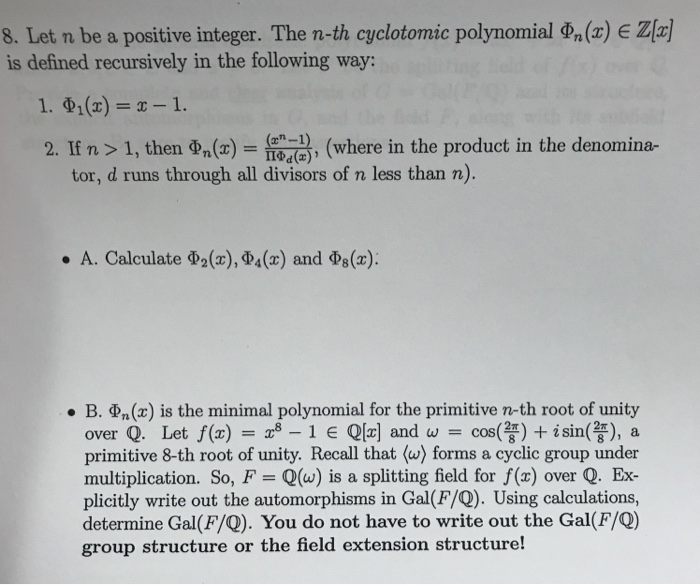8. Let n be a positive integer. The n-th cyclotomic polynomial Ф,a(z) E Z is defined recursively in the following way: 1. Ф1(x)-x-1. 2. If n > 1, then Фп(x)- , (where in the product in the denomina- tor, d runs through all divisors of n less than n). . A. Calculate Ф2(x), Ф4(x) and Ф8(z): . B. n(x) is the minimal polynomial for the primitive n-th root of unity over Q. Let f(x) = "8-1 E Q[a] and ω...

• ### 2. Calculate the inverse Fourier transform of X(cfw) = {2 2j 0 <W <T -2j -n<w...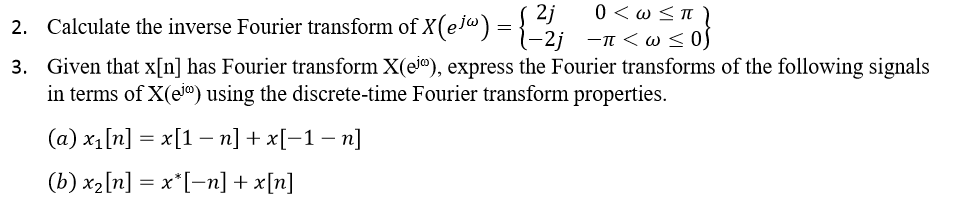2. Calculate the inverse Fourier transform of X(cfw) = {2 2j 0 <W <T -2j -n<w < 3. Given that x[n] has Fourier transform X(@j®), express the Fourier transforms of the following signals in terms of X(el“) using the discrete-time Fourier transform properties. (a) x1[n] = x[1 – n] + x[-1 - n] (b) x2 [n] = x*[-n] + x[n]

• ### roblem 3: (15-7+8 points) Consider the left-sided discrete-time signal a(n)42+1). a) Find the discrete Fourier transform...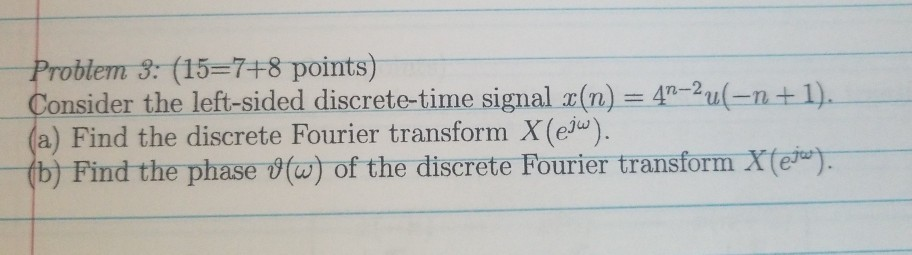roblem 3: (15-7+8 points) Consider the left-sided discrete-time signal a(n)42+1). a) Find the discrete Fourier transform X(eju n-2 ). (b) Find the phase (o) of the discrete Fourier transform X

• ### numerical analysis problem 4. Let s = (2,1, -4,3). Find the discrete Fourier transform F(s) of...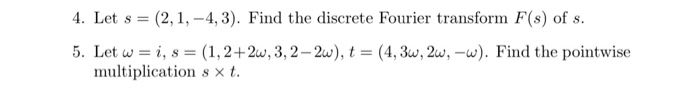numerical analysis problem 4. Let s = (2,1, -4,3). Find the discrete Fourier transform F(s) of s. 5. Let w=i, s = (1, 2+2w, 3, 2-2w), t = (4,3w, 2w, -w). Find the pointwise multiplication ext.

• ### A sequence has the discrete-time Fourier transform 1 - a2 X(e) ae-jw)2(1- aejw) la| < 1...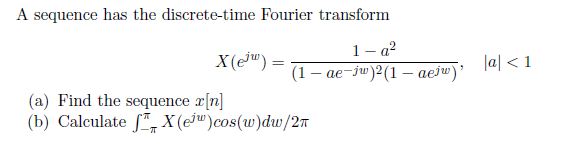A sequence has the discrete-time Fourier transform 1 - a2 X(e) ae-jw)2(1- aejw) la| < 1 (a) Find the sequence r[n] (b) Calculate X(eju)cos(w)dw/27

• ### QUESTION 2 [25 Marks Determine the Fourier Transform, H(2), of the discrete impulse response h[n]. where...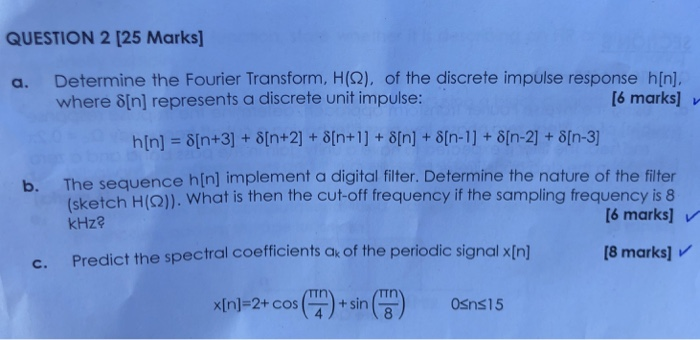QUESTION 2 [25 Marks Determine the Fourier Transform, H(2), of the discrete impulse response h[n]. where ?[n] represents a discrete unit impulse: a. [6 marks] h[n] ?[n+3] + ?[n+2] + ?[n+1 ] + ?[n] + ?[n-1 ] + ?[n-2] + ?[n-3] The sequence h[n] implement a digital filter. Determine the nature of the filter sketch H(Q)). What is then the cut-off frequency if the sampling frequency is 8 kHz? b. [6 marks] v c. Predict the spectral coefficients a of...

• ### 10ρ 18ρ A signal (t) has the Fourier transform X(jw) indicated in the figure. The signal is sampled to obtain the discrete time signal 1. Sketch the Fourier transform Xr(jw) of x[n] for T-to. 2. Can...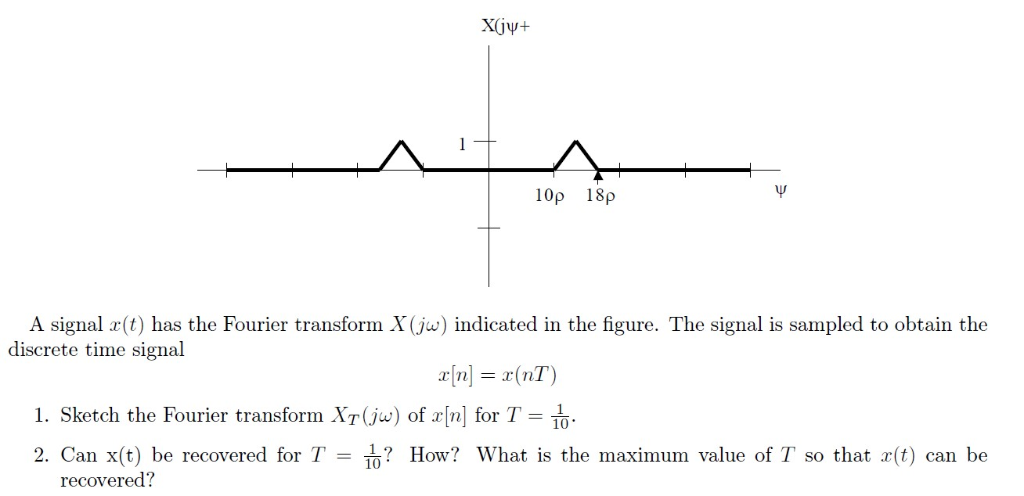10ρ 18ρ A signal (t) has the Fourier transform X(jw) indicated in the figure. The signal is sampled to obtain the discrete time signal 1. Sketch the Fourier transform Xr(jw) of x[n] for T-to. 2. Can x(t) be recovered for T? How? What is the maximum value of T so that r(t) can be recovered? 10ρ 18ρ A signal (t) has the Fourier transform X(jw) indicated in the figure. The signal is sampled to obtain the discrete time signal 1....

• ### 6. (16 points) Let CE C be a primitive n-th root of unity. Let X =...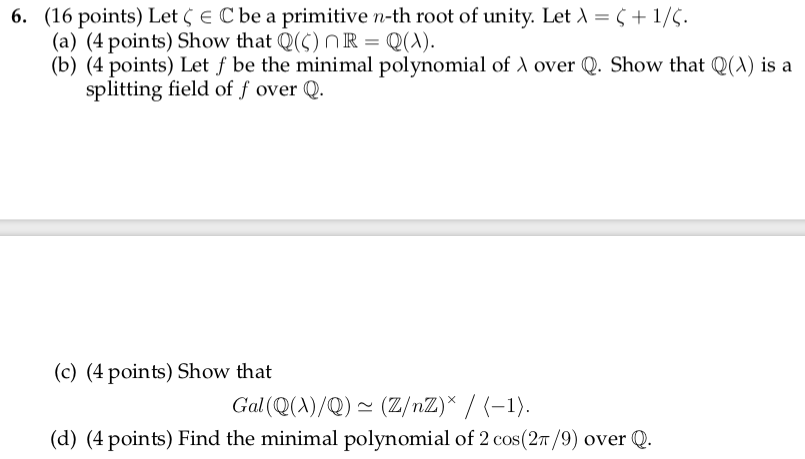6. (16 points) Let CE C be a primitive n-th root of unity. Let X = 6 + 1/5. (a) (4 points) Show that Q(5) R = Q(1). (b) (4 points) Let f be the minimal polynomial of over Q. Show that Q(x) is a splitting field of f over Q. (c) (4 points) Show that Gal(Q(^)/Q) – (Z/nZ)* / (-1). (d) (4 points) Find the minimal polynomial of 2 cos(27/9) over Q.

Free Homework App